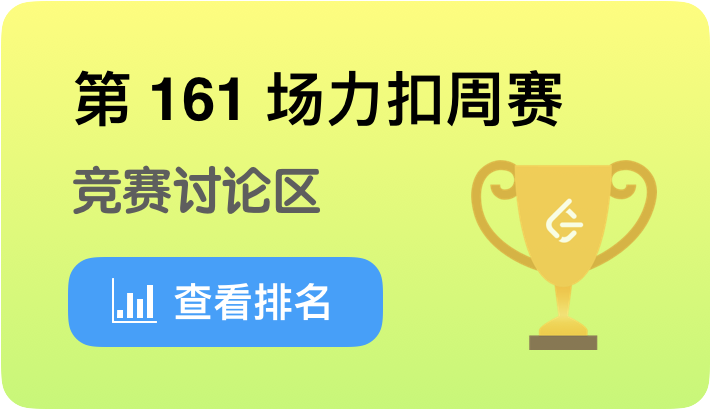🏆 第 161 场力扣周赛4 分 - 交换字符使得字符串相同
4 分 - 统计「优美子数组」
5 分 - 移除无效的括号
6 分 - 检查「好数组」

• 交换字符使得字符串相同
统计位置的匹配情况，一共只有4种可能，$x-x$,$x-y$,$y-x$,$y-y$，其中1和4是相同的情况，可以不管。我们统计两个字符串有多少对$x-y$ 以及 $y-x$
然后，我们可以根据匹配情况的统计进行贪心，如果我们同时有两对 $x-y$ 或者 $y-x$，是可以通过一次交换使这两对相同的，最后会剩下只有一对不相同，这种情况是 $-1$；或者各有一对不相同，这时我们需要用两次操作将他们变成一样。总体时间复杂度为$O(n)$

• 统计「优美子数组」
遍历数组，记录从 $0$ 位置到当前位置的奇数数量。我们用一个dict来记录历史的数量的总和，然后对于每个位置，如果我们知道到这个位置有 $x$ 个奇数，那么只需要去dict里查 $x-k$ 个奇数的数量就是以当前位置结尾的子数组数量了。总体时间复杂度为$O(n)$

• 移除无效的括号
经典的合法括号序列问题，只是这题需要我们记录方案。
还是用一个栈维护括号状态，对于左括号，入栈，对于右括号，如果栈中有左括号，左括号出栈，否则右括号不记入答案。
最后再把左括号栈中剩余的左括号从答案中删除就可以了，总体时间复杂度$O(n)$

• 检查「好数组」
唯一的结论是如果数组中所有数的最大公约数为 $1$，则存在解，否则不存在。所以只需要计算所有数最大公约数即可，时间复杂度$O(nlog(m))$，其中 $m$ 为数字大小。

7